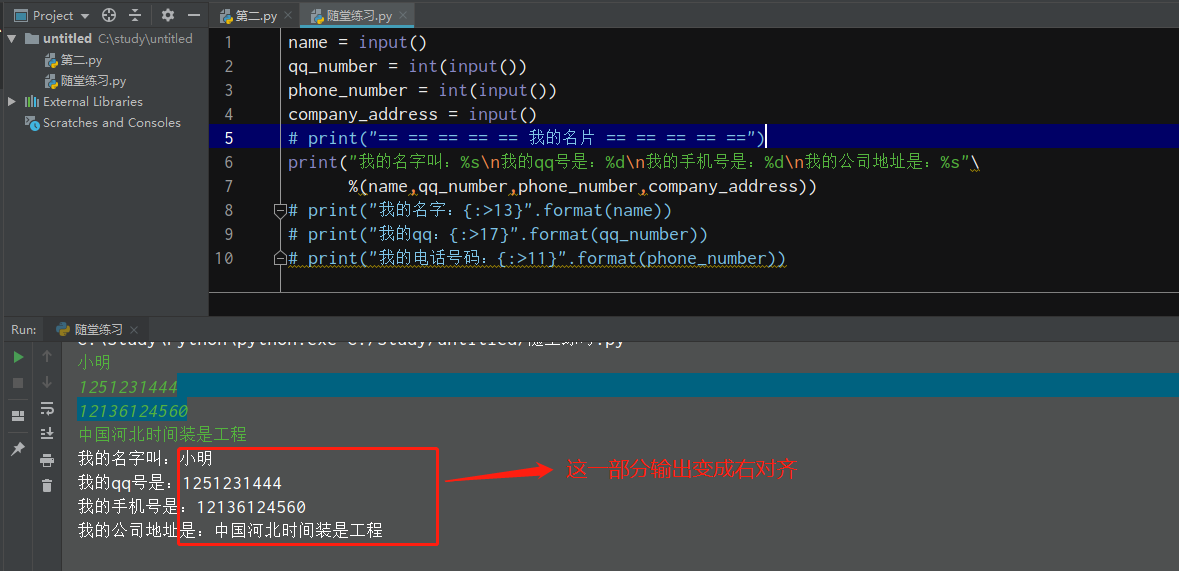python3中 如何在print输出中将输出的数据右对齐

``````name = input()
qq_number = int(input())
phone_number = int(input())
````````````print("我的名字叫：%6s\n我的qq号是：%6d\n我的手机号是：%6d\n我的公司地址是：%s"\
print("我的名字：{:>13}".format(name))
print("我的qq：{:>17}".format(qq_number))
print("我的电话号码：{:>11}".format(phone_number))
``````

1个回答

``````print("
我的名字叫：\t\t%s\n
我的qq号是：\t\t%s\n
我的手机号是：\t\t%s\n
我的公司地址是：\t%s\n"